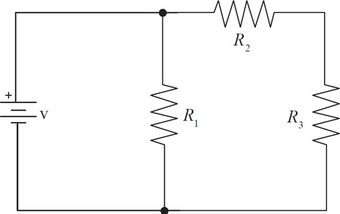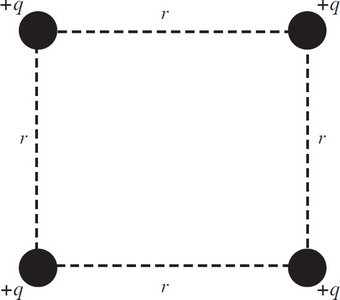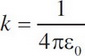# AP Physics 1 Practice Test 5

### Test Information10 questions18 minutes

1. A stopper of mass M is tied to a string of length R and swings around horizontally in uniform circular motion with a velocity of v when acted on by a force of F.

What is the period of the stopper?

2. A stopper of mass M is tied to a string of length R and swings around horizontally in uniform circular motion with a velocity of v when acted on by a force of F.

What is the power developed by the force?

3. Two objects, A and B, have equal charge and equal mass. Neither body is in motion because the gravitational and electrostatic forces between them are equal. If the mass of Object A is halved, equilibrium will be maintained if

4. Which configuration of battery and resistors will create a circuit with the greatest current?

5. Which of the following changes to a circuit will always bring about an increase in the current?

1.If R1 were to burn out, the current coming out from the battery would

2. Wire #1 has three times the length and twice the diameter of Wire #2. If both wires are made of the same material and if R1 is the resistance of Wire #1 and R2 is the resistance of Wire #2, then which of the following is true?

3.A Wheatstone bridge (diagram above) is a configuration of resistors and a sensitive current meter, called a galvanometer, that is used to determine the resistance of an unknown resistor. In the Wheatstone bridge shown here, find the value of Rx such that the current through galvanometer G is zero.

4. If T, C, M, and L represent the dimensions of time, charge, mass, and length, respectively, which of the following represents the correct dimensions for the permittivity of free space (ε0)?

5.In the figure above, four charges are arranged. If the magnitudes of all the charges q are all the same and the distance R between them is as shown above, what is the magnitude of the net force on the bottom right charge in terms of q, R, and k (where)?